If you have an Excel sheet with a lot of dates, chances are you will eventually need to calculate the differences between some of those dates. Maybe you want to see how many months it took you to pay off your debt or how many days it took you to lose a certain amount of weight?

Calculating the difference between dates in Excel is easy, but can become complicated depending on how you want to calculate certain values. For example, if you wanted to know the number of months between 2/5/2016 and 1/15/2016, should the answer be 0 or 1? Some people might say 0 since it’s not a full month between the dates and others might say 1 because it’s a different month.

In this article, I’ll show you how to calculate the differences between two dates to find the number of days, months and years with different formulas, depending on your preferences.

## Days Between Two Dates

The simplest calculation we can do is to get the number of days between two dates. The good thing about calculating days is that there really is only one way to calculate the value, so you don’t have to worry about different formulas giving you different answers.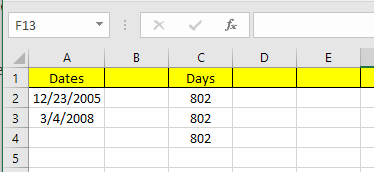In my example above, I have two dates stored in cells A2 and A3. At the right, you can see the difference between those two dates is 802 days. In Excel, there are always multiple ways to calculate the same value and that’s what I did here. Let’s take a look at the formulas: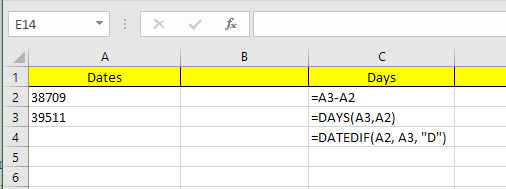The first formula is just a simple subtraction of the two dates, A3 – A2. Excel knows it’s a date and simply calculates the number of days between those two dates. Easy and very straight-forward. In addition, you can also use the DAYS function.

`=DAYS(A3, A2)`

This function takes two arguments: the end date and the start date. If you switch the dates in the formula, you’ll just get a negative number. Lastly, you can use a function called DATEDIF, which is included in Excel from Lotus 1-2-3 days, but isn’t an officially supported formula in Excel.

`=DATEDIF(A2, A3, "D")`

When you type the formula, you’ll see that Excel does not give you any suggestions for the fields, etc. Luckily, you can see the syntax and all supported arguments for the DATEDIF function here.

As an added bonus, what if you wanted to calculate the number of weekdays between two dates? That’s easy enough also because we have a built-in formula:

`=NETWORKDAYS(startDate,endDate)`

Calculating the number of days and weekdays is simple enough, so let’s talk about months now.

## Months Between Two Dates

The trickiest calculation is the number of months because of how you can either round up or round down depending on whether it’s a complete month or a partial month. Now there is a MONTHS function in Excel, but it’s very limited because it will only look at the month when calculating the difference and not the year. This means it’s only useful for calculating the difference between two months in the same year.

Since that is kind of pointless, let’s look at some formulas that will get us the correct answer. Microsoft has provided these here, but if you are too lazy to visit the link, I have provided them below also.

`Round Up - =(YEAR(LDate)-YEAR(EDate))*12+MONTH(LDate)-MONTH(EDate)`
```Round Down - =IF(DAY(LDate)>=DAY(EDate),0,-1)+(YEAR(LDate)-YEAR(EDate))
*12+MONTH(LDate)-MONTH(EDate)```

Now these are two fairly long and complicated formulas and you really don’t need to understand what’s going on. Here are the formulas in Excel: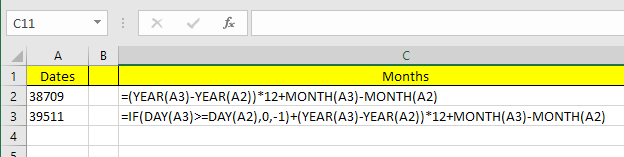Note the you should edit the round down formula in the actual cell itself because for some odd reason the entire formula does not show up in the formula bar. In order to see the formula in the cell itself, click on the Formulas tab and then click Show Formulas.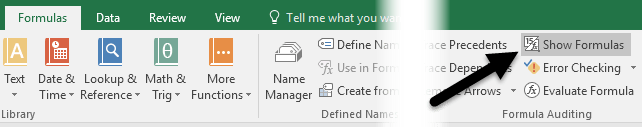So what’s the final result of the two formulas on our current example? Let’s see below: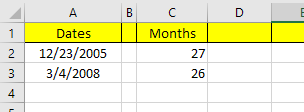Round up gives me 27 months and round down gives me 26 months, which is accurate, depending on how you want to look at it. Lastly, you can also use the DATEDIF function, but it only calculates full months, so in our case the answer it returns is 26.

`=DATEDIF(A2, A3, "M")`

## Years Between Two Dates

As with months, years can also be calculated depending on whether you want to count 1 year as a full 365 days or if a change in the year counts. So let’s take our example where I have used two different formulas for calculating the number of years: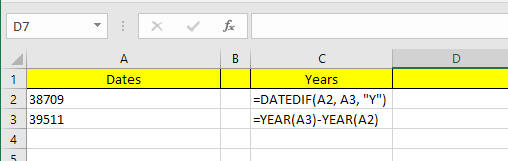One formula uses DATEDIF and the other uses the YEAR function. Since the difference in the number of days is only 802, DATEDIF shows 2 years whereas the YEAR function shows 3 years.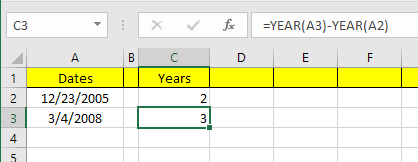Again, this is a matter of preference and depends on what exactly you are trying to calculate. It’s a good idea to know both methods so that you can attack different problems with different formulas.

As long as you are careful with which formulas you use, it’s fairly easy to calculate exactly what you are looking for. There are also a lot more date functions outside of the ones I mentioned, so feel free to check those out also on the Office Support site. If you have any questions, feel free to comment. Enjoy!